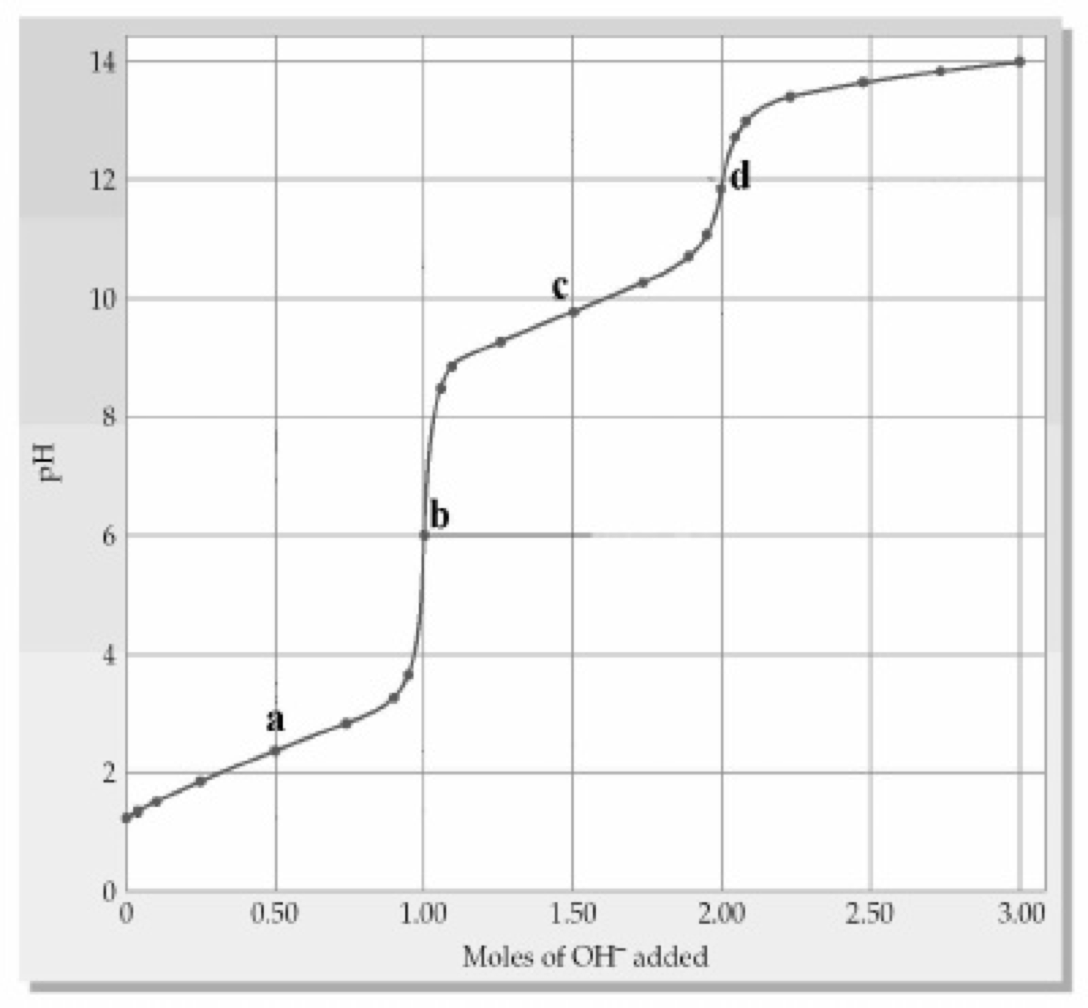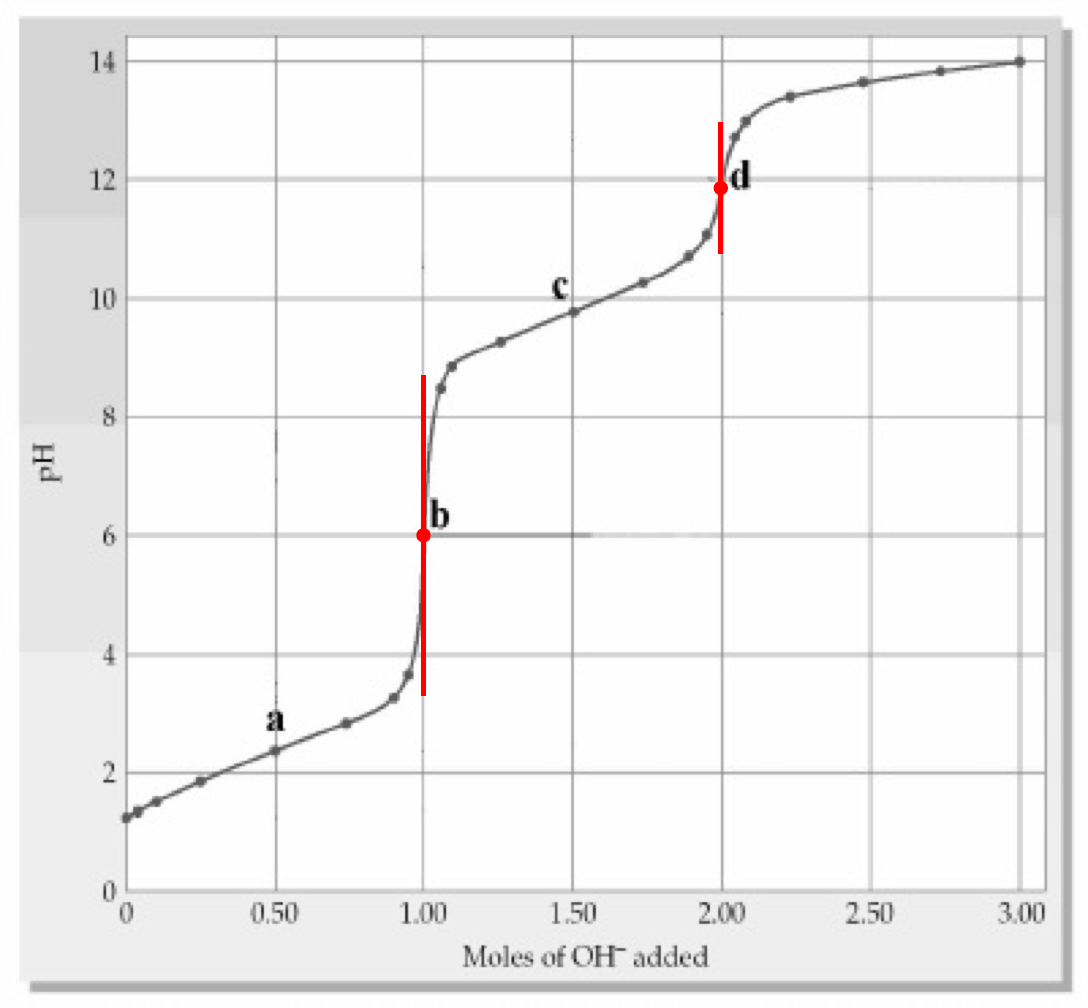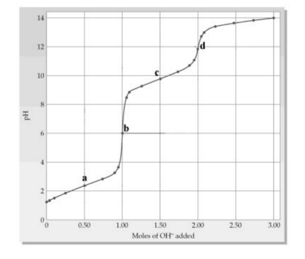# Problem: The following plot below shows a titration curve for the titration of 1.00 L of 1.00 M diprotic acid H2A with NaOH. Which point(s) a-d represent(s) the H 2X/HX - buffer region?(a) point a(b) point b(c) point c(d) point d(e) points a and c

###### FREE Expert Solution
90% (147 ratings)
###### FREE Expert Solution

We’re being asked to determine which point(s) a–d represent(s) the H2X/HX– buffer region, given the following titration curve for the titration of 1.00 L of 1.00 M diprotic acid H2A with NaOH:Since H2A is a diprotic acid, this means it has two equivalence points

Recall that the equivalence point is the point in a titration curve where the pH increases abruptly. In the given titration curve, the two equivalence points are represented by points b and d:90% (147 ratings)###### Problem Details

The following plot below shows a titration curve for the titration of 1.00 L of 1.00 M diprotic acid H2A with NaOH. Which point(s) a-d represent(s) the H 2X/HX - buffer region?

(a) point a

(b) point b

(c) point c

(d) point d

(e) points a and cWhat scientific concept do you need to know in order to solve this problem?

Our tutors have indicated that to solve this problem you will need to apply the Titrations of Diprotic and Polyprotic Acids concept. You can view video lessons to learn Titrations of Diprotic and Polyprotic Acids. Or if you need more Titrations of Diprotic and Polyprotic Acids practice, you can also practice Titrations of Diprotic and Polyprotic Acids practice problems.

What is the difficulty of this problem?

Our tutors rated the difficulty ofThe following plot below shows a titration curve for the tit...as medium difficulty.

How long does this problem take to solve?

Our expert Chemistry tutor, Jules took 3 minutes and 33 seconds to solve this problem. You can follow their steps in the video explanation above.

What professor is this problem relevant for?

Based on our data, we think this problem is relevant for Professor O'Connor's class at CSU OHIO.